Courses

# RD Sharma Solutions -Ex-23.1, Data Handling Bar Graphs, Class 6, Maths Class 6 Notes | EduRev

## RD Sharma Solutions for Class 6 Mathematics

Created by: Abhishek Kapoor

## Class 6 : RD Sharma Solutions -Ex-23.1, Data Handling Bar Graphs, Class 6, Maths Class 6 Notes | EduRev

The document RD Sharma Solutions -Ex-23.1, Data Handling Bar Graphs, Class 6, Maths Class 6 Notes | EduRev is a part of the Class 6 Course RD Sharma Solutions for Class 6 Mathematics.
All you need of Class 6 at this link: Class 6

Q. 1. The following table shows the daily production of T.V sets in an industry for 7 days of a week:

 Days Number of Tv sets Mon 300 Tue 400 Wed 150 Thurs 250 Fri 100 Sat 350 Sun 200

Represent the above information by a pictograph.

Sol:

Let an icon of a T.V represent 50 T.Vs.

Then, the number of icons produced by the industry on different days of a week is as follows:

 Days Number of icons Mon 300 / 50 = 6 Tue 400 / 50 = 8 Wed 150 / 50 = 3 Thurs 250 / 50 = 5 Fri 100 / 50 = 5 Sat 350 / 50 = 7 Sun 200 / 50 = 4

The pictograph representing the above data is as follows: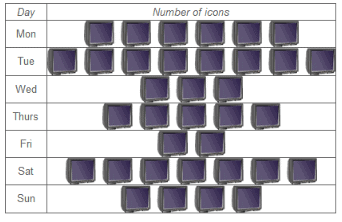Q. 2. The following table shows the number of Maruti cars sold by five dealers in a particular month:

 Dealer Cars sold Saya 60 Bagga links 40 D.D Motors 20 Bhasin Motor 15 Competent motor 10

Sol:

Let one car icon represent 5 Maruti cars.

Then, the numbers of icons sold by the five dealers in a particular month are as follows:

 Dealer Number of icons Saya 60 / 5 = 12 Bagga links 40 / 5 = 8 D.D Motors 20 / 5 = 4 Bhasin Motor 15 / 5 = 3 Competent motor 10 / 5 = 3

The pictograph representing the above data is as follows:

 Dealer Number of icons Saya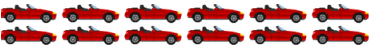Bagga links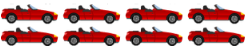D.D Motors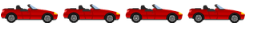Bhasin Motor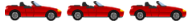Competent motor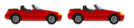Q. 3. The population of Delhi state in different census years is as given below:

 Census year 1961 1971 1981 1991 2001 Population in lakhs 30 55 70 110 150

Represents the above information by a pictograph.

Sol:

To represent the given data on a bar graph, we should first draw a horizontal and a vertical line. Here, the horizontal line will show the census year and the vertical line will show represent the population.

Since 5 values or data are given, we mark 5 points on the horizontal axis at equal distances and will erect rectangles of the same width with their heights proportional to the given data.

Also, on the vertical axis, each difference of 10 will represent a population of 10 lakhs.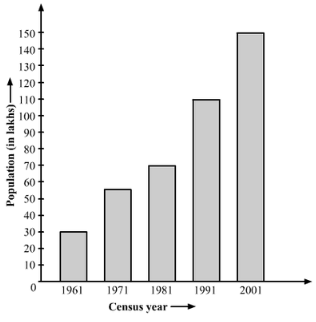Q. 4. Read the bar graph shown in Fig 23.8 and answer the following question: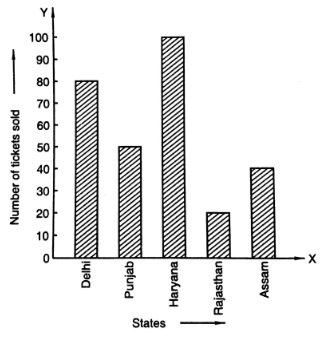(i) What is the information given by the bar graph?

(ii) How many tickets of Assam States Lottery were sold by the agent?

(iii) Of which state, where the maximum number of tickets sold?

(iv) State whether true or false

The maximum number of tickets sold is three times the minimum number of tickets sold

(v) of which states were the minimum number of tickets sold.

Sol:

(i) The bar graph represents the number of tickets of different states lotteries sold by an agent on a single day.

(ii) The agent sold 40 tickets of Assam state lottery.

Explanation: The vertical height of the rectangle against Assam, on the bar graph, has ended on the 40thmark against the vertical axis.

(iii) Haryana

Explanation: The vertical height of the rectangle against Haryana I the maximum compared to those against the other states.

(iv) False

Explanation: Maximum vertical length (against the state of Haryana) = 100 units

Minimum vertical length (against the state of Rajasthan) = 20 units

Therefore, Maximum number of lottery sold for one state = 100 tickets

Minimum number of lottery tickets sold for one state = 20 tickets

(v) Rajasthan

Explanation: From the bar graph we can say that the Rajasthan State Lottery tickets were sold the minimum i.e. only 20 tickets.

Q.5. Study the bar graph representing the number of persons in various age groups in a town shown in Fig 23.9. observe the bar graph and answer the following questions: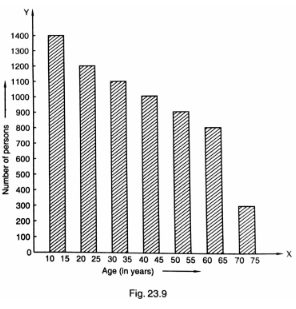(i) What is the percentage of the youngest age – group persons over those in the oldest age group?

(ii) What is the total population of the town?

(iii) What is the number of persons in the age group 60 – 65?

(iv) How many persons are more in the age – group 10 -15 than in the age group 30 – 35?

(v) What is the age – group of exactly 1200 persons living in the town?

(vi) What is the total number of persons living in the town in the age – group 50 – 65?

(vii) What is the total number of persons living in the town in the age – group 10 – 15 and 60 – 65?

(viii) Whether the population in general increases decreases or remains constants with the increase in the age – group.

Sol:

(i) The youngest age group is 10 – 15 years.

Number of persons in the youngest age group = 1400

The oldest age group is 70 – 75 years.

Number of persons in the oldest age group = 300

Difference in the number of people in the youngest age oldest age group = 1400 – 300 = 1100

Therefore, The youngest group has 1100 more people than the oldest group.

Therefore, % of the youngest group over oldest group

1100300×100% = 11003% = 36623%

(ii) Total population of the town

= Total number of people from all age groups

= 1400 + 1200 + 1100 + 1000 + 900 + 800 + 300 = 6700.

(iii) There are 800 persons in the age group 60 – 65 years.

Explanation: The vertical length of the rectangle against the age group 60 – 65is up to 800 units.

(iv) Number of persons in the age group 10 – 15 = 1400

Number of persons in the age group 30 – 35 = 1100

Therefore, number of more persons in the age group 10 – 15 as compared to that in the age group 30 – 35 = 1400 – 1100 = 300.

(v) the age – group of exactly 1200 people living in the town is 20 – 25 years.

Explanation: Looking at the bar graph we can say that the vertical length of the rectangle against the age group 20 – 25 is up to 1200 units.

(vi) The number of people of the age group 50 – 55 years is 900.

Explanation: The vertical length of the rectangle against the age group 50 – 55 years is up to 900 units.

(vii) The number of persons in the age group 10 – 15 years is 1400, and that in the age group 60 – 65 years is 800.

Therefore, Total number of persons in the age group 10 – 15 years and 60 – 65 years = 1400 + 800 = 2200

(viii) With the increase in the age group, the population decreases.

Explanation: As the age group increases, the heights of the rectangle start falling.

Q. 6. Read the bar graph shown in fig 23.10 and answer the following questions:

(i) What is the information given by the bar graph?

(ii) What was the number of commercial banks in 1977?

(iii) What is the ratio of the number of commercial banks in 1969 to that in 1980?

(iv) State whether true or false:

The number of commercial banks in 1983 is less than double the number of commercial banks in 1969.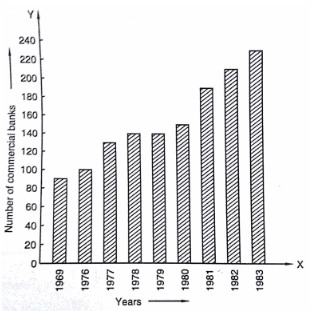Sol:

(i) The bar graph represents the number of commercial banks in India during the respective years.

(ii) In 1977, there were 130 commercial banks.

Explanation: The height of the rectangle against the year 1977 is up to 130 units.

(iii) Number of commercial banks in 1969 = 90

Number of commercial banks in 1980 = 150

Therefore, Ratio of the number of commercial banks in 1969 to that in 1980 =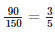=3:5

(iv) False

Explanation: Number of commercial banks in 1983 = 230

Number of commercial banks in 1969 = 90

Therefore,2×90=180

As 230 is greater than 180, the number of commercial banks in 1983 is not less than double the number of commercial banks in 1969.

Q. 7. Given below is the bar graph indicating the marks obtained out of 50 in mathematics paper by 100 students. Read the bar graph and answer the following questions: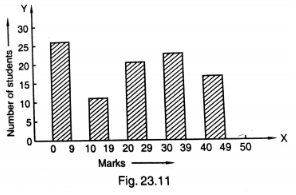(i) It is decided to distribute workbooks on mathematics to the students obtaining less than 20 marks, giving one workbook to each of such students. If a workbook costs Rs. 5, what sure is required to buy the workbooks?

(ii) Every student belonging to the highest mark group is entitled to get a prize of Rs. 10. How much amount of money is required for distributing the prize money?

(iii) Every student belonging to the lowest mark-group has to solve 5 problems per day. How many problems, in all, will be solved by the students of this group per day?

(iv) State whether true or false.

(a) 17% students have obtained marks ranging from 40 to 49.

(b) 59 students have obtained marks ranging from 10 to 29.

(v) What is the number of students getting less than 20 marks?

(vi) What is the number of students getting more than 29 marks?

(vii) What is the number of students getting marks between 9 and 40?

(Viii) What is the number, of students belonging to the highest mark group?

(ix) What is the number of students obtaining more than 19 marks?

Sol:

Let us prepare a chart of the 100 students using the data from the bar graph.

 Marks Number of students 0 – 9 27 10 – 19 12 20 – 29 20 30 – 39 24 40 – 49 17

(i) Number of students with less than 20 marks = 27 + 12 = 39

Therefore, Required sum to buy the workbooks = Rs 5 x 39 = Rs 195.

(ii) Highest marks group = 40 – 49

Number of students in this marks group = 17

Therefore, Required money to distribute the prize = Rs 10 x 17 = Rs 170.

(iii) Lowest marks group = 0 – 9

Number of students in this marks group = 27

Therefore, Number of problems that will be solved by the students per day = 5 x 27 = 135.

(iv) (a) True, (b) False

(v) Number of students scoring less than 20 marks.

= Number of students in the marks group 0 – 9 + Number of students in the marks group 10 – 19 = 27 + 12 = 39

(vi) Number of students scoring more than 29 marks

= Number of students in the marks group 30 – 39 + number of students in the marks group 40 – 49 = 24 +17 = 41

(vii) Number of students scoring between 9 and 40

= Number of students in the marks group 10 — 19 + Number of students in the marks group 20 — 29 + Number of students in the marks group 30 — 39

= 12 + 30 + 24 = 56

(viii) The highest marks group is 40 — 49.

Number of students in this marks group = 17

(ix) Number of students scoring more than 19 marks

= Number of students in the marks group 20 — 29 + Number of students in the marks group 30 — 39 + Number of students in the marks group 40 — 49

= 20 + 24 + 17 = 61

Q. 8. Read the following bar graph (Fig 23.12) and answer the following questions: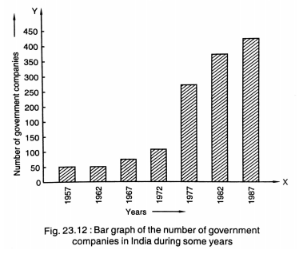(i) What is the information given by the bar graph?

(ii) State each of the following whether true or false.

(a) The number of government companies in 1957 is that of 1982 is 1: 9.

(b) The number of government companies has decreased over the year 1957 to 1983.

Sol: (i) The bar graph represents the number of government companies in India from 1957 to 1987.

(ii) (a) False

Explanation: Number of government companies in 1957 = 50

Number of government companies in 1982 = 375

The ratio of the number of government companies in 1957 to that in 1982 =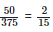= 2:15

(b) False

Explanation: As no data is given for the year 1983, we cannot tell anything about this. Hence, the given statement is false.

Q. 9. Read the following bar graph and answer the following questions: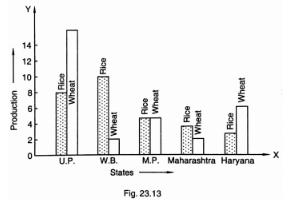(i) What information is given by the bar graph?

(ii) Which state is the largest producer of rice?

(iii) Which state is the largest producer of wheat?

(iv) Which state has total production of rice and wheat as its maximum?

(v) Which state has the total production of wheat and rice minimum?

Sol: Let’s draw a chart using the data from the above bar graph:

 States Rice Production Wheat Production Total Production U.P 8 16 24 W.B 10 2 12 M.P 5 5 10 Maharashtra 4 2 6 Haryana 3 6 9

(i) The above bar graph provides information on the production of rice and wheat in various states of India.

(ii) W.B. is the largest producer of rice.

Explanation: The height of the rectangle representing rice production in W.B. is up to 10 units, i.e., the highest compared to those in the other states.

(iii) U.P. is the largest producer of wheat.

Explanation: The height of the rectangle representing wheat production in U.P. is up to 16 units, i.e., the highest compared to those in the other states.

(iv) U.P. has the total production of rice and wheat as its maximum.

Explanation: From the bar graph we can say that U.P. exceeds the other states in the total production of rice and wheat, i.e., 16 units wheat + 8 units of rice = 24 units.

(v) Maharashtra has the total production of rice and wheat as its minimum.

Explanation: From the bar graph we can say that the total production of rice and wheat in Maharashtra is the minimum, i.e., 4 units rice + 2 units of wheat = 6 units.

Q. 10. The following bar graph represents the heights (in cm) of 50 students of class XI of a particular school. Study the graph and answer the questions: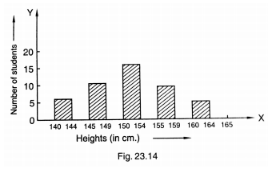(i) What percentage of the total number of students have their heights more than 149 cm?

(ii) How many students in the class are in the range of maximum height of the class?

(iii) The school wants to provide a particular type of tonic to each student below the height of 150 cm to improve his height. If the cost of the tonic for each student comes out to be Rs 55, how much amount of money is required?

(iv) How many students are in the range of shortest height of the class?

(v) State whether true or false:

(a) There are 9 students in the class whose heights are in the range of 155-159 cm.

(b) Maximum height (in cm) of a student in the class is 17.

(c) There are 29 students in the class whose heights are in the range of 145-154 cm.

(d) Minimum height (in cm) of a student is the class is in the range of 140-144 cms.

(e) The number of students in the class having their heights less than150 cm is 12.

(f) There are 14 students each of whom has height more than 154 cm.

Sol:

Let’s draw a chart based on the above bar graph:

 Heights (in cm) Number of students 140 – 144 7 145 – 149 12 150 – 154 17 155 – 159 9 160 – 164 5

(i) Number of students whose height is more than 149 cm = 17 + 9 + 5 = 31

Total number of students = 50

Percentage of students whose height is more than 149 cm =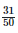% = 31 x 2% = 62%

(ii) The maximum height-range of the class is 160 — 164. Number of students in this range = 5

(iii) Number of students measuring less than 150 cm = 7 + 12 = 19 Required amount of money to be spent for the tonic = 19 x Rs. 55 = Rs. 1045

(iv) The minimum height-range of the class is 140 — 144 Number of students in this range = 7

(v) (a) True

Explanation: From the above chart we can say that the number of students in the height-range 155 — 159 is 9.

(b) False

Explanation: 17 is the number of students found in the maximum height-range, i.e., 160 — 164.

(c) True

Explanation: Number of students in the class whose heights are in the range of 145 — 154 cm = Number of students in the class whose heights are in the range of 145 — 149 + Number of students in the class whose heights are in the range of 150 — 154 = 12 + 17 = 29

(d) True

Explanation: The minimum height-range of the students in the class is 140 — 144 cm.

(e) False

Explanation: Number of students measuring less than 150 cm = 7 + 12 = 19

(f) True

Explanation: Number of students measuring more than 154 cm = 9 + 5 = 14

Q. 11. Read the following bar graph (Fig. 23.15) and answer the following questions: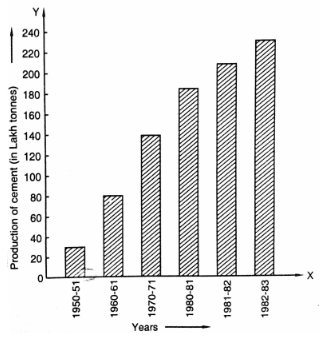(i) What is the information given by the bar graph?

(ii) What was the production of cement in the year 1980 – 81?

(iii) What are the minimum and maximum productions of cement and corresponding years?

Sol:

(i) The bar graph informs us about the industrial production of cement in India in different financial years.

(ii) 186 lakh tones of cement were produced in the year 1980 – 81.

Explanation: The height of the bar against the year 1980 — 81 is up to186 units, and production of cement is shown in lakh tones.

(iii) Minimum height of a bar is 30 units against the year 1950 — 51.

Therefore, Minimum production of cement is 30 lakh tonnes in the year 1950 — 51.

The maximum height of a bar is 232 units against the year 1982 — 83.

Maximum production of cement is 232 lakh tonnes in the year 1982 — 83.

Q. 12. The bar graph shown in fig 23.16 represents the circulation of newspapers in 10 languages. Study the graph and answer the following questions: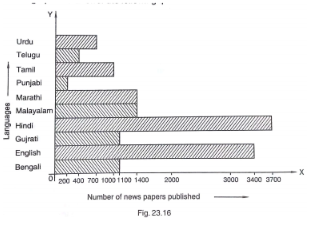(i) What is the total number of newspapers published in Hindi, English, Urdu, Punjabi, and Bengali?

(ii) What percent is the number of newspapers published in Hindi of the total number of newspapers?

(iii) Find the excess of the number of newspapers published in English over those published in Urdu.

(iv) Name two pairs of languages which publish the same number of newspapers.

(v) State the language, in which the smallest number of newspapers is published,

(vi) State the language in which the largest number of newspapers is published.

(vii) State the language in which the number of newspapers published is between 2500 and 3500.

(viii) State whether true or false:

(a) The number of newspapers published in Malayalam and Marathi together is less than those published in English.

(b) The number of newspapers published in Telugu is more than those published in Tamil.

Sol:

Let’s draw a chart using the information from the above bar graph:

 Language Number of newspapers published Urdu 700 Telugu 400 Tamil 1000 Punjabi 200 Marathi 1400 Malayalam 1400 Hindi 3700 Gujarati 1100 English 3400 Bengali 1100

(i) Total number of newspapers published in Hindi, English, Urdu, Punjabi and Bengali = 3700 + 3400 + 700 + 200 + 1100 = 9100

(ii) Number of newspaper published in Hindi = 3700

Total number of newspapers published = 700 + 400 + 1000 + 200 + 1400 + 1400 + 3700 + 1100 + 3400 + 1100 = 14400

Therefore, Percentage of Hindi newspaper published =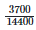×100%%

= 25.69%

= 25.7%

(iii) Number of newspapers published in English = 3400

Number of newspapers published in Urdu = 700

Therefore, Excess number of the newspapers published in English over Urdu = 3400 — 700 = 2700

(iv) "Marathi" & Malayalam" and "Gujarati & Bengali' are the two pairs of languages in which same number of newspapers are published.

Explanation: Newspapers published in Marathi = Newspapers published in Malayalam = 1400

Newspapers published in Gujarati = Newspapers published in Bengali = 1100

(v) Punjabi is the language in which the least number of newspapers are published.

Explanation: In Punjabi, only 200 newspapers are published.

(vi) Hindi is the language in which the maximum numbers of newspapers are published.

Explanation: In Hindi, 3700 newspapers are published.

(vii) The number of English newspapers published is between 2500 and 3500.

Explanation: In English, 3400 newspapers are published.

(viii)

(a) True

Explanation: Total number of newspapers published in Malayalam and Marathi = 1400 + 1400 = 2800

The number of newspapers published in English are 3400, which is more than the total numbers of Malayalam and Marathi newspapers.

(b) False Explanation: Number of newspapers published in Telugu = 400

Number of newspapers published in Tamil = 1000

103 docs

,

,

,

,

,

,

,

,

,

,

,

,

,

,

,

,

,

,

,

,

,

,

,

,

,

,

,

,

,

,

;